• 图的割点 在一个无向连通图中，如果删除某个顶点后，图不再连通（即任意两点之间不能相互到达），我们称这样的顶点为割点（或者称割顶）。 上图中的2号顶点就是割点，因为删除2号后，4,5不通，1,6也不通。   ...
图的割点

在一个无向连通图中，如果删除某个顶点后，图不再连通（即任意两点之间不能相互到达），我们称这样的顶点为割点（或者称割顶）。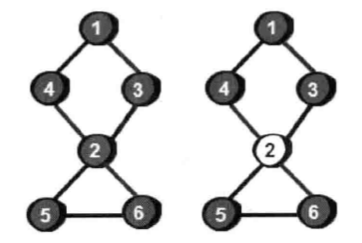上图中的2号顶点就是割点，因为删除2号后，4,5不通，1,6也不通。

很容易想到的方法是：依次删除每一个顶点，然后用dfs或者bfs来检查图是否依然连通。如果删除某个顶点后，导致图不再连通，那么刚才删除的顶点就是割点。

这种方法的时间复杂度是O(N(N+M))。

下面寻找复杂度低的方法来解决。

dfs遍历上图后，如下图，圆圈中数字是顶点编号，圆圈右上角的数表示这个顶点在遍历时是第几个被访问到的，叫做“时间戳”。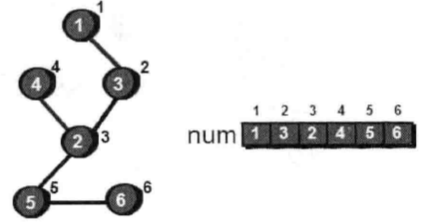基本思路：

假如我们在dfs时访问到了u点，此时图就会被u点分割成为两部分。一部分是已经被访问过的点，另一部分是没有被访问过的点。如果u点是割点，那么剩下的没有被访问过的点中至少有一个点在不经过u点的情况下，是无论如何再也回不到已经访问过的点了。假如到了u后，图中还有顶点v是没有访问过的点，如何判断v在不经过u的情况下是否还能回到之前访问过的任意一个点？u是v的父亲，而之前访问过的顶点就是祖先。也就是如何检测v在不经过父亲u的情况下还能否回到祖先。那就是对v再进行一次dfs，但此次遍历不经过u，看能否回到祖先。不能u即为割点。

我们需要一个数组low来记录每个顶点在不经过父顶点时，能够回到的最小“时间戳”。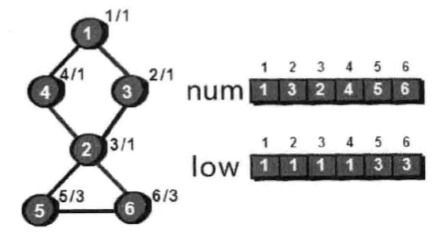对于某个顶点u，如果存在至少一个顶点v（u的儿子），使得low[v]>=num[u]，即不能回到祖先，那么u点为割点。

以上转自：https://blog.csdn.net/wtyvhreal/article/details/43530613

下面来一道模板题：洛谷-割边

直接上代码：

#include<iostream>
#include<cstdio>
#include<cstring>
#include<algorithm>
#include<cstdlib>
#include<vector>
using namespace std;

const int maxn = 100005;
vector <int> G[maxn];
int low[maxn];//数组low来记录每个顶点在不经过父顶点时，能够回到的最小时间戳
int flag[maxn];//记录割点集
int dfn[maxn];//dfn记录每个点的dfs序的序号
int dfs_clock;//进行时间截的递增
int cnt;//记录割点个数
void dfs(int u, int f){//点编号和父节点的编号
int child = 0;
dfn[u] = low[u] = ++dfs_clock;
for(int i = 0; i < G[u].size(); i++){
int v = G[u][i];
if(!dfn[v])//时间截为零，说明没被访问过
{
dfs(v, u);
child++;
low[u] = min(low[v], low[u]);//更新当前顶点cur能否访问到最早顶点的时间截
if(low[v] >= dfn[u] && f != 0) flag[u] = 1;//v往回找到的最大时间截所在点还在u的子树里，则为u割点
}
else{
low[u] = min(low[u], dfn[v]);//如果顶点u曾经被访问过，更新u的low值
}
}
if(f == 0 && child == 1) flag[u] = 0;//源点就一条出边，一定不是割点
if(flag[u])cnt ++;
}

int main(){
int n,m;
scanf("%d%d", &n,&m);
memset(dfn, 0, sizeof(dfn));
memset(low, 0x3f3f3f3f, sizeof(low));
dfs_clock = 0;
for(int i = 0; i <= n; i++) G[i].clear();
int h, t;
for(int i = 0; i < m; i++){
scanf("%d%d", &h,&t);
G[h].push_back(t);
G[t].push_back(h);//无向图
}
cnt = 0;
for(int i = 1; i <= n; i++){
if(!dfn[i])
dfs(i, 0);
}
printf("%d\n", cnt);
for(int i = 1; i <= n; i++){
if(flag[i]) printf("%d ",i);
}
return 0;
}


展开全文• 无向图的割点、割边 Tarjan算法求无向图的割点 //啊哈算法 图的割点 #include <cstdio> #include <algorithm> #include <cstring> using namespace std; typedef long long ll; const int N = 2...
无向图的割点和桥

在一个无向图中，如果有一个顶点集合，删除这个顶点集合以及这个集合中所有顶点相关联的边以后，图的连通分量增多，就称这个点集为割点集合。
如果某个割点集合只含有一个顶点X（也即 {X} 是一个割点集合），那么X称为一个割点。

设G是一个图，x是G的一条边，如果G-x的连通分支数大于G的连通分支数，则称x是G的一个桥，或割边。

双连通分量又分点双连通分量和边双连通分量两种。
若一个无向图中的去掉任意一个节点（一条边）都不会改变此图的连通性，即不存在割点（桥），则称作点（边）双连通图。一个无向图中的每一个极大点（边）双连通子图称作此无向图的点（边）双连通分量。
求双连通分量可用Tarjan算法。

点双连通分量和边双连通分量

若一个无向图中的去掉任意一个节点都不会改变此图的连通性，即不存在割点，则称作点双连通图。一个无向图中的每一个极大点双连通子图称作此无向图的点双连通分量。
注意一个割点属于多个点双连通分量。

若一个无向图中的去掉任意一条边都不会改变此图的连通性，即不存在桥，则称作边双连通图。一个无向图中的每一个极大边双连通子图称作此无向图的边双连通分量。
连接两个边双连通分量的边即是桥。
边双连通分量：可将其看做一棵树或者森林，节点为边双连通分量，树边为桥。

边双连通分量和点双连通分量的区别与联系

二者都是基于无向图
边双连通分量是删边后还连通，而后者是删点
点双连通分量一定是边双连通分量（除两点一线的特殊情况），反之不一定
点双连通分量可以有公共点，而边双连通分量不能有公共边

代码
Tarjan算法求无向图的割点
邻接表存图，输入为 $n$ 、$m$ 表示 $n$ 个点 $m$ 条边，接着输入 $m$ 条边，输出第一行为割点的个数，然后一行为割点的编号。
#include <iostream>
#include <algorithm>
#include <cstdio>
#include <vector>
#include <cstring>

using namespace std;
const int N = 2e4 + 10;

int n, m, root;
vector<int> e[N];    //邻接表存图
int num[N], low[N], idx;
bool flag[N];

void dfs(int cur, int father) {
int child = 0;  //child记录cur儿子的数量
idx++;  //时间戳
num[cur] = idx; //cur的时间戳
low[cur] = idx; //cur能访问到最早顶点的时间戳，开始时是自己
int es = e[cur].size();
for (int i = 0; i < es; ++i) {
int v = e[cur][i];
if (num[v] == 0) {
child++;
dfs(v, cur);    //i作为子节点，cur作为父节点，继续往下深度优先遍历
low[cur] = min(low[cur], low[v]);
if (cur != root && low[v] >= num[cur]) {
flag[cur] = true;
}
if (cur == root && child == 2) {
flag[cur] = true;
}
} else if (v != father) {
low[cur] = min(low[cur], num[v]);
//为什么不写 low[cur] = min(low[cur], low[i]); https://blog.csdn.net/elijahqi/article/details/80614953
}
}
}

int main() {
scanf("%d%d", &n, &m);
memset(flag, 0, sizeof(flag));
for (int i = 1; i <= m; ++i) {
int x, y;
scanf("%d%d", &x, &y);
e[x].push_back(y);
e[y].push_back(x);
}
for (int i = 1; i <= n; ++i) {
if (num[i] == 0) {
root = i;
dfs(i, root);
}
}
int ans = 0;
for (int i = 1; i <= n; ++i) {
if (flag[i]) {
ans++;
}
}
printf("%d\n", ans);
for (int i = 1; i <= n; ++i) {
if (flag[i]) {
printf("%d ", i);
}
}
printf("\n");
return 0;
}

Tarjan算法求无向图的割边（桥）
邻接矩阵存图。
//啊哈算法 图的割边
#include <cstdio>
#include <algorithm>
#include <cstring>

using namespace std;
typedef long long ll;
const int N = 2e4 + 10;

int n, m, root;
bool e[N][N];	//邻接矩阵存图
int num[N], low[N], idx;

void dfs(int cur, int father) {
idx++;  //时间戳
num[cur] = idx; //cur的时间戳
low[cur] = idx; //cur能访问到最早顶点的时间戳，开始时是自己
for (int i = 1; i <= n; ++i) {
if (e[cur][i] == 1) {
if (num[i] == 0) {
dfs(i, cur);	//i作为子节点，cur作为父节点，继续往下深度优先遍历
low[cur] = min(low[cur], low[i]);
if (low[i] > num[cur]) {	//不能回到祖先，也没有另一条路能回到父亲，为割边
printf("%d-%d\n", cur, i);
}
} else if (i != father) {
low[cur] = min(low[cur], num[i]);  //为什么不写 low[cur] = min(low[cur], low[i]); 参见博客 https://blog.csdn.net/elijahqi/article/details/80614953
}
}
}
}

int main() {
int x, y;
scanf("%d%d", &n, &m);
memset(e, 0, sizeof(e));
for (int i = 1; i <= m; ++i) {
scanf("%d%d", &x, &y);
e[x][y] = e[y][x] = true;
}
//如果给出的无向图是连通的
root = 1;
dfs(1, root);
/*
如果给出的无向图不一定连通
for (int i = 1; i <= n; ++i) {
if (num[i] == 0) {
root = i;
dfs(i, root);
}
}
*/
return 0;
}

时间复杂度

实际应用中需要用邻接表或链式前向星存图，这样的话以上两个算法（Tarjan求割点割边）的时间复杂度为O(N+M)。

疑问
更新low值时为什么不写 low[cur] = min(low[cur], low[i]) 而是写 low[cur] = min(low[cur], num[i])
详见博客 https://blog.csdn.net/elijahqi/article/details/80614953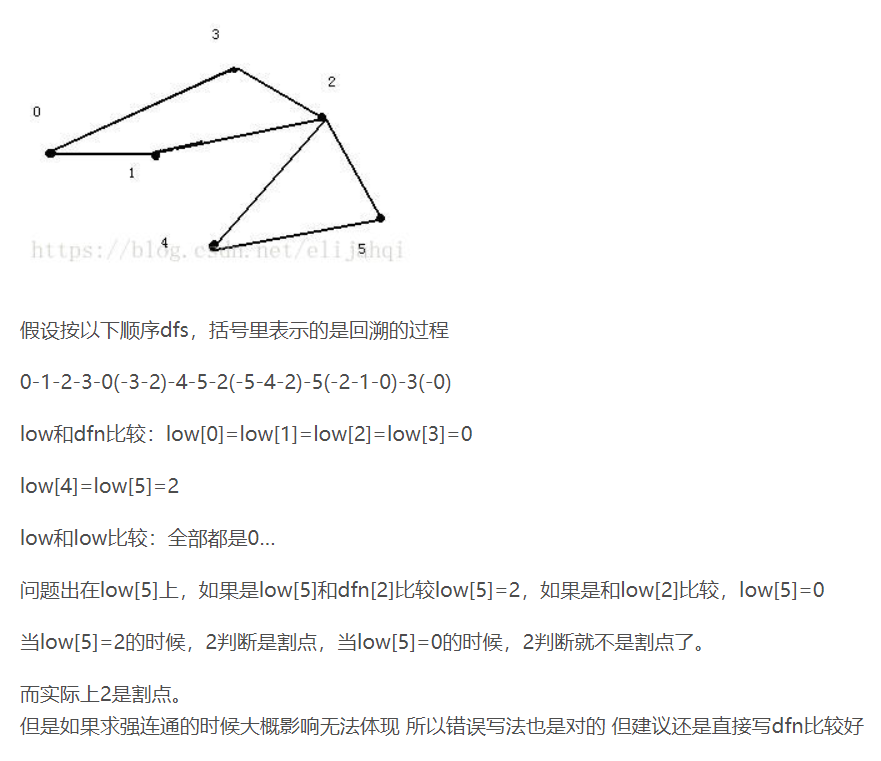展开全文• tarjan算法求无向图的割点与桥 一篇tarjan算法求割点与桥的完整的解释,写的真的好认真 以下代码来自kuangbin的模板 4.5 图的割点、桥和双连通分支的基本概念 [点连通度与边连通度] 在一个无向连通图中，如果有一...
tarjan算法求无向图的割点与桥
一篇tarjan算法求割点与桥的完整的解释,写的真的好认真

以下代码来自kuangbin的模板

4.5 图的割点、桥和双连通分支的基本概念
[点连通度与边连通度] 在一个无向连通图中，如果有一个顶点集合，删除这个顶点集合，以
及这个集合中所有顶点相关联的边以后，原图变成多个连通块，就称这个点集为割点集合。
一个图的点连通度的定义为，最小割点集合中的顶点数。
类似的，如果有一个边集合，删除这个边集合以后，原图变成多个连通块，就称这个点集为
割边集合。一个图的边连通度的定义为，最小割边集合中的边数。
[双连通图、割点与桥]
如果一个无向连通图的点连通度大于1，则称该图是点双连通的(point biconnected)，简称
双连通或重连通。一个图有割点，当且仅当这个图的点连通度为1，则割点集合的唯一元素
被称为割点(cut point)，又叫关节点(articulation point)。
如果一个无向连通图的边连通度大于1，则称该图是边双连通的(edge biconnected)，简称双
连通或重连通。一个图有桥，当且仅当这个图的边连通度为1，则割边集合的唯一元素被称
为桥(bridge)，又叫关节边(articulation edge)。
可以看出，点双连通与边双连通都可以简称为双连通，它们之间是有着某种联系的，下文中
提到的双连通，均既可指点双连通，又可指边双连通。
[双连通分支]
在图G 的所有子图G’ 中，如果G’ 是双连通的，则称G’ 为双连通子图。如果一个双连
kuangbin 138
ACM Template of kuangbin
通子图G’ 它不是任何一个双连通子图的真子集，则G’ 为极大双连通子图。双连通分支
(biconnected component)，或重连通分支，就是图的极大双连通子图。特殊的，点双连通分
支又叫做块。[求割点与桥]
该算法是R.Tarjan 发明的。对图深度优先搜索，定义DFS(u) 为u 在搜索树（以下简称为
树）中被遍历到的次序号。定义Low(u) 为u 或u 的子树中能通过非父子边追溯到的最早的
节点，即DFS 序号最小的节点。根据定义，则有：
Low(u)=Min DFS(u) DFS(v) (u,v) 为后向边(返祖边) 等价于DFS(v)<DFS(u) 且v 不为u
的父亲节点Low(v) (u,v) 为树枝边(父子边) 一个顶点u 是割点，当且仅当满足(1) 或(2)
(1) u 为树根，且u 有多于一个子树。(2) u 不为树根，且满足存在(u,v) 为树枝边(或称父子
边，即u 为v 在搜索树中的父亲)，使得DFS(u)<=Low(v)。
一条无向边(u,v) 是桥，当且仅当(u,v) 为树枝边，且满足DFS(u)<Low(v)。
[求双连通分支]
下面要分开讨论点双连通分支与边双连通分支的求法。
对于点双连通分支，实际上在求割点的过程中就能顺便把每个点双连通分支求出。建立一个
栈，存储当前双连通分支，在搜索图时，每找到一条树枝边或后向边(非横叉边)，就把这条
边加入栈中。如果遇到某时满足DFS(u)<=Low(v)，说明u 是一个割点，同时把边从栈顶一
个个取出，直到遇到了边(u,v)，取出的这些边与其关联的点，组成一个点双连通分支。割点
可以属于多个点双连通分支，其余点和每条边只属于且属于一个点双连通分支。
对于边双连通分支，求法更为简单。只需在求出所有的桥以后，把桥边删除，原图变成了多
个连通块，则每个连通块就是一个边双连通分支。桥不属于任何一个边双连通分支，其余的
边和每个顶点都属于且只属于一个边双连通分支。
[构造双连通图]
一个有桥的连通图，如何把它通过加边变成边双连通图？方法为首先求出所有的桥，然后删
除这些桥边，剩下的每个连通块都是一个双连通子图。把每个双连通子图收缩为一个顶点，
再把桥边加回来，最后的这个图一定是一棵树，边连通度为1。
统计出树中度为1 的节点的个数，即为叶节点的个数，记为leaf。则至少在树上添加
(leaf+1)/2 条边，就能使树达到边二连通，所以至少添加的边数就是(leaf+1)/2。具体方
法为，首先把两个最近公共祖先最远的两个叶节点之间连接一条边，这样可以把这两个点到
祖先的路径上所有点收缩到一起，因为一个形成的环一定是双连通的。然后再找两个最近公
共祖先最远的两个叶节点，这样一对一对找完，恰好是(leaf+1)/2 次，把所有点收缩到了一
起。

/*
* 求无向图的割点和桥
* 可以找出割点和桥，求删掉每个点后增加的连通块。
* 需要注意重边的处理，可以先用矩阵存，再转邻接表，或者进行判重
*/
const int MAXN = 10010;
const int MAXM = 100010;
struct Edge {
int to, next;
bool cut;//是否为桥的标记
}edge[MAXM];
int head[MAXN], tot;
int Low[MAXN], DFN[MAXN];

int Stack[MAXN];
int Index, top;
bool Instack[MAXN];

bool cut[MAXN];//每个顶点是否为割的标记
int add_block[MAXN];//删除一个点后增加的连通块

int bridge;
void addedge(int u, int v) {
edge[tot].to = v;
edge[tot].next = head[u];
edge[tot].cut = false;
head[u] = tot++;
}

//当前顶点u,u的父亲节点pre
void Tarjan(int u, int pre) {
int v;
Low[u] = DFN[u] = ++Index;
Stack[top++] = u;
Instack[u] = true;

//儿子节点
int son = 0;
int pre_cnt = 0; //处理重边，如果不需要可以去掉
for (int i = head[u]; i != −1; i = edge[i].next) {
v = edge[i].to;
//v是u的父亲节点并且重边的个数为1,重边的个数++
//上面说明找到了一条重边, 处理重边
if (v == pre && pre_cnt == 0) { pre_cnt++; continue; }
//第一种情况, 下一条边未被DFS过
//son++
//递归Tarjan
//更新low[]数组
//low[u]=min(low[u],low[v])
//low[i]代表顶点i不经过父节点可以达到的最早的祖先节点
if (!DFN[v]) {
son++;
Tarjan(v, u);
if (Low[u] > Low[v])Low[u] = Low[v];
//桥
//一条无向边(u,v) 是桥，当且仅当(u,v) 为树枝边，且满足DFS(u) < Low(v)。
if (Low[v] > DFN[u]) {
bridge++;
edge[i].cut = true;
edge[i ^ 1].cut = true;
}
//割点
//一个顶点u 是割点，当且仅当满足(1) 或(2) (1) u 为树根，且u有多于一个子树。
//(2) u 不为树根，且满足存在(u,v) 为树枝边(或称父子边，
//即u 为v 在搜索树中的父亲)，使得DFS(u)<=Low(v)

//u是割点, 当且仅当u不是第一个DFS的节点(根节点)并且其某个子树节点无法通过u遍历到u的祖先节点
if (u != pre && Low[v] >= DFN[u]) {//不是树根
cut[u] = true;
add_block[u]++;
}
}
//找到祖先节点
else if (Low[u] > DFN[v])
Low[u] = DFN[v];
}
//树根，分支数大于1, 必然是割点
if (u == pre && son > 1)cut[u] = true;
//增加的联通块的个数
if (u == pre)add_block[u] = son - 1;
Instack[u] = false;
top--;
}



展开全文• 对于无向连通图G=(V,E)，Tarjan算法可以在线性时间内求出无向图的割点和桥。 割点：对于x∈V，如果删除某个点x，图的联通分量增多（G分裂为两个或者两个以上的子图），则x为割点。 桥 ：对于e∈E，如果删除e，G...
定义

对于无向连通图G=(V,E)，Tarjan算法可以在线性时间内求出无向图的割点和桥。

割点：对于x∈V，如果删除某个点x，图的联通分量增多（G分裂为两个或者两个以上的子图），则x为割点。

桥    ：对于e∈E，如果删除e，G分裂成两个不相连的子图，则称e为桥或割边。

时间戳（T[i]）：DFS的过程中，第一次访问到节点的时间，将N个节点依次标记为1-N。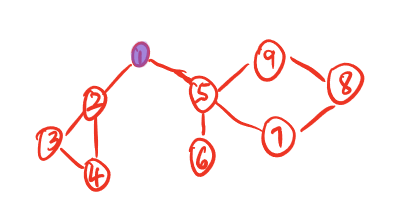搜索树：按照DFS的顺序建树。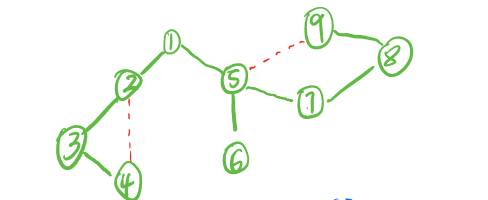追溯值（low[i]）：low[i] = min(T[i通过不在搜索树上的边到达的点] , i的子树中的节点)。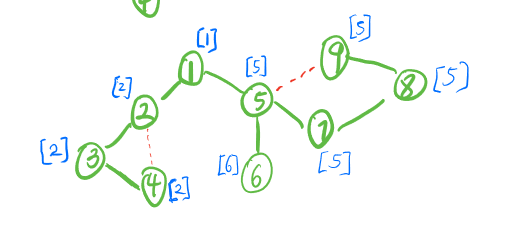割边判定法则：当节点x的子节点y满足T[x] < low[y] 时，边（x，y）为桥。  证明：当T[x] < T[y] 时 y走除了（x，y）外的任意边都无法到达时间戳更小的节点。

桥一定是搜索树中的边，简单环中的边一定都不是桥。

模板（完美支持重边 powered by 李煜东）

#include <bits/stdc++.h>
using namespace std;

const int maxn = 1e5+10;
int dfn[maxn],low[maxn],n,num,tot,m;
int head[maxn],ver[maxn<<1],Next[maxn<<1];
bool bridge[maxn<<1];

void add(int x , int y)
{
ver[++tot] = y,Next[tot]=head[x],head[x] = tot;
}
void Tarjan(int x, int in_edge)
{
dfn[x] = low[x] =  ++num;
for(int i = head[x] ; i ; i = Next[i])
{
int y = ver[i];
if(!dfn[y])
{
Tarjan(y,i);
low[x] = min(low[x],low[y]);

if(low[y] > dfn[x])
{
bridge[i] = bridge[i^1] = true;
}
}
else if(i != (in_edge^1))
{
low[x] = min(low[x] , dfn[y]);
}
}
}

int main()
{
cin >> n >> m;
//    num = 0;
tot = 1;
for(int i = 0 ; i < m ; i++)
{
int x,y;
cin >> x >> y;
add(x,y);add(y,x);
}

for(int i = 1 ; i <= n ; i++)
{
if(!dfn[i])
Tarjan(i,0);
}

for(int i = 2 ; i < tot ; i += 2 )
{
if(bridge[i])printf("%d %d\n",ver[i^1],ver[i]);
}
}



展开全文• 无向图的割点和桥 割点和桥的概念: 对于无向图G,如果删除某个点u后,连通分量数目增加(本来为一个连通集，现在变为多个)，称u为图的割点。对于连通图，割点就是删除之后不再连通的点。当然了，桥也同理，只不过桥是边...
• * 求 无向图的割点和桥 * 可以找出割点和桥，求删掉每个点后增加的连通块。 * 需要注意重边的处理，可以先用矩阵存，再转邻接表，或者进行判重 * 调用solve输出割点数，全局变量bridge记录边的个数 */ #include #...
• 无向图的割点和桥的模板，大白书312页内容，用邻接表存储，为dfs添加时间戳这个概念，同时递归计算low函数的值 模板如下 int dfs(int u, int fa) { int lowu = pre[u] = ++dfs_clock; int child = 0;//子节点...
• 桥：是存在于无向图这样一条边，如果去掉这一条边，那么整张无向图会分为两部分，这样一条边称为桥无向连通图中，如果删除某边后，图变成不连通，则称该边为桥。 割点：无向连通图中，如果删除某点后，图...
• 无向图的割点，也称关节点。对于无向图中不同的两点u，v，如果必须经过点w，才能构成一条从u到v的路径，那么称该w点就是割点（关节点）。关节点的求解只需要一次关于图的深度优先遍历（完成一次DFS等于生成一棵树，...DFS
• 1. 无向图的割点求法：利用Tarjan算法思想，若一个点为割点，那么只存在两种情况：（1）该点是根节点，且有两个以上子节点（2）该点不上根节点，但是该点的低位数大于等于DFS数低位数的定义：从该顶点v出发，只用...
• 割点是将其删除后会破坏连通性顶点，割点存在表示该不是双连通。 #include <queue> #include <iostream> #include <algorithm> using namespace std; const int maxn = 100; int ...
• 无向图的割点&&点双联通分量(Tarjan算法) 在一个无向图中，对于一个点对（u，v），如果从u至少有两条点不重复路径到达v，那么点u和点v在同一个点双联通分量中。而一个点双联通分量即为包含了尽可能多的这样的（u，v...Tarjan算法
• ...如果将连通图G中的某个点及和这个点相关的边删除后，将使原图不再连通，那么这个点就称为图G的割点或是接合点。如果一个无向图没有割点，则这样的图被称为双连通图。关于图的割点，有如下两条性质：
• 同求有向图的强连通分量类似，都是使用到了dfn以及low数组用来标记搜索树的状态。但有所区别的是，在深搜的时候要区别根的情况以及非根结点，如果是根只要有两个或两个以上的子树分支这个肯定是割点；而对于非根结点...
• 　给定无向图G=（V，E）： 　若对于x∈V，从图中删去节点x以及所有与节点x相关联的边后，G分裂成两个或两个以上不相连的子图，则称x为G的割点。 　若对于e∈E，从图中删去边e后，G分裂成两个不相连的子图，则称e为...
• 判断一个点u(令其儿子为v)是否为割点： 1. u==root:他子节点数cnt>1 2. u!=root:low[u]>=dfn[v] //因为如果low[u]<dfn[v] 则说明u点一定有边可以回溯到v前面形成一个环。 */ #include <cstdio> #...
• Tarjan无向图的割点和桥(割边) 导言 在掌握这个算法前,咱们有几个先决条件. [x] DFS搜索 [x] DFS序 [x] 一张纸 [x] 一支笔 [x] 认真的大脑(滑稽) 如果您都具备了,那么您就是巨佬了,您就可以轻松解决Tarjan算法了. ......